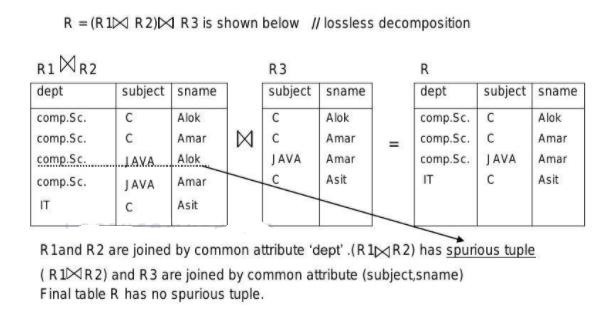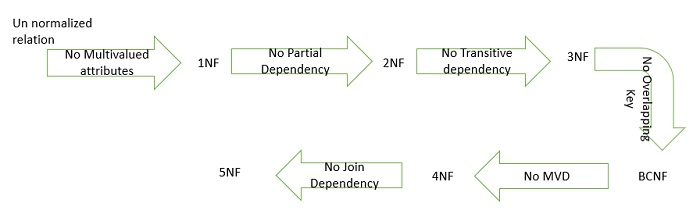# Explain 5NF with examples in DBMS

It is also called as project join normal form. A relation R is in 5NF if it is in 4NF and there is no join dependency. No join dependency means lossless-join decomposition.

Decomposition is lossless-join decomposition if it preserves all the data in original relation and does not result in additional tuples.

A relation R satisfies join dependency iff R is equal to the join of R1,R2,.....Rn where Ri are a subset of the set of attributes of R.

Relation R

DeptSubjectName
CSECAmmu
CSECAmar
CSEJavaAmar
ITCbhanu

Here,

dept ->-> subject

dept->-> name

The above relation is in 4NF. Anomalies can occur in relation in 4NF if the primary key has three or more fields. The primary key is (dept,subject, name). Sometimes decomposition of a relation into two smaller relations does not remove redundancy. In such cases it may be possible to decompose the relation in three or more relations using 5NF.

The above relation says that dept offers many elective subjects which are taken by a variety of students. Students have the opinion to choose subjects. Therefore, all three fields are needed to represent the information.

The above relation does not show non-trivial MVDs since the attributes subject and name are dependent; they are related to each other (A FD subject->name exists). The relation cannot be decomposed in two relations (dept, subject) and (dept,sname).

Therefore the relation can be decomposed into following three relations −

R1(dept, subject)

R2(dept, name) and

R3(subject, name) and it can be shown that decomposition is lossless.

R1

DeptSubject
CSEC
CSEJava
ITC

R2

DeptName
CSEAmmu
CSEAmar
ITBhanu

R3

SubjectName
CAmmu
CAmar
JavaAmar
CBhanu

In above decomposition of table reduction of redundancy is not apparent, but it can be realized when tables contain large amounts of data.Let's have a look at all Normal forms can be understood in single picture as shown below −